# Spectral geometry of Riemannian submersions

Let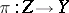be a Riemannian submersion. Letand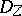be operators of Laplace type (cf. also Laplace operator) onand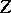on bundlesand. Let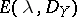and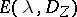be the corresponding eigenspaces. Assume given a pull-back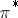from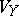to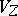. One wants to have examples where there exists(a1)

One also wants to know when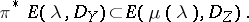(a2)

Let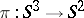and let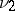be the volume element on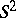. Let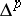be the Laplace–Beltrami operator (cf. also Laplace–Beltrami equation). Y. Muto [a8], [a7] observed that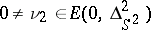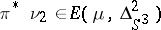for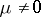; he also gave other examples involving principal fibre bundles.

S.I. Goldberg and T. Ishihara [a2] and B. Watson [a9] studied this question and determined some conditions to ensure that (a2) holds with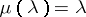for all; this work was later extended in [a5] for the real Laplacian and in [a3] for the complex Laplacian. If (a1) holds for a single eigenvalue, then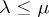(eigenvalues cannot decrease). See also [a1] for a discussion of the case in which the fibres are totally geodesic. See [a6] for related results in the spin setting. For a survey of the field, see [a4].